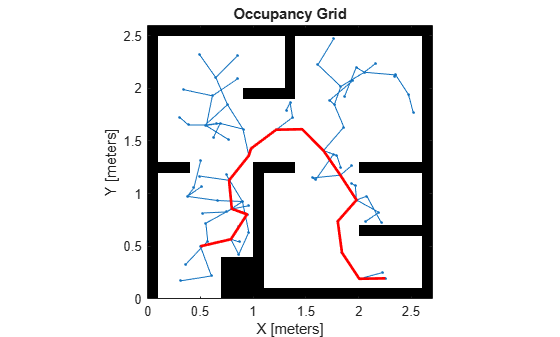# stateSpaceSE2

SE(2) state space

## Description

The `stateSpaceSE2` object stores parameters and states in the SE(2) state space, which is composed of state vectors represented by [x, y, θ]. x and y are Cartesian coordinates, and θ is the orientation angle. The object uses Euclidean distance to calculate distance and uses linear interpolation to calculate translation and rotation of the state.

## Creation

### Syntax

``space = stateSpaceSE2``
``space = stateSpaceSE2(bounds)``

### Description

example

````space = stateSpaceSE2` creates an SE(2) state space object with default state bounds for x, y, and θ.```
````space = stateSpaceSE2(bounds)` specifies the `bounds` for x, y, and θ. The state values beyond the bounds are truncated to the bounds. The input, `bounds`, allows you to set the value of the StateBounds property.```

## Properties

expand all

Name of state space, specified as a string.

Dimension of the state space, specified as a positive integer.

Bounds of state variables, specified as a 3-by-2 real-valued matrix.

• The first row specifies the lower and upper bounds of the x state in meters.

• The second row specifies the lower and upper bounds of the y state in meters.

• The third row specifies the lower and upper bounds of the θ state in radians.

Data Types: `double`

Weight applied to x and y distance calculation, specified as a nonnegative real scalar.

In the object, the distance calculated as:

`$d=\sqrt{\left({w}_{xy}\left({d}_{x}^{2}+{d}_{y}^{2}\right)\right)+{w}_{\theta }{d}_{\theta }^{2}}$`

wxy is weight applied to x and y coordinates, and wθ is the weight applied to the θ coordinate. dx, dy, and dθ are the distances in the x, y, and θ direction, respectively.

Data Types: `double`

Weight applied to θ distance calculation, specified as a nonnegative real scalar.

In the object, the distance calculated as:

`$d=\sqrt{\left({w}_{xy}\left({d}_{x}^{2}+{d}_{y}^{2}\right)\right)+{w}_{\theta }{d}_{\theta }^{2}}$`

wxy is weight applied to x and y coordinates, and wθ is the weight applied to the θ coordinate. dx, dy, and dθ are the distances in the x, y, and θ direction, respectively.

Data Types: `double`

## Object Functions

 `copy` Create deep copy of state space object `distance` Distance between two states `enforceStateBounds` Reduce state to state bounds `interpolate` Interpolate between states `sampleGaussian` Sample state using Gaussian distribution `sampleUniform` Sample state using uniform distribution

## Examples

collapse all

Create an SE(2) state space.

`ss = stateSpaceSE2;`

Create an `occupancyMap`-based state validator using the created state space.

`sv = validatorOccupancyMap(ss);`

Create an occupancy map from an example map and set map resolution as 10 cells/meter.

```load exampleMaps map = occupancyMap(simpleMap,10); sv.Map = map;```

Set validation distance for the validator.

`sv.ValidationDistance = 0.01;`

Update state space bounds to be the same as map limits.

`ss.StateBounds = [map.XWorldLimits;map.YWorldLimits; [-pi pi]];`

Create the path planner and increase maximum connection distance.

```planner = plannerRRT(ss,sv); planner.MaxConnectionDistance = 0.3;```

Set the start and goal states.

```start = [0.5,0.5,0]; goal = [2.5,0.2,0];```

Plan a path with default settings.

```rng(100,'twister'); % for repeatable result [pthObj,solnInfo] = planner.plan(start,goal);```

Visualize the results.

```map.show; hold on; plot(solnInfo.TreeData(:,1),solnInfo.TreeData(:,2),'.-'); % tree expansion plot(pthObj.States(:,1), pthObj.States(:,2),'r-','LineWidth',2) % draw path```